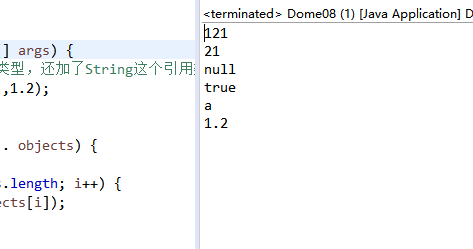# 面相對像（基礎）## 方法的可變參數：

```public class Dome {
/* 方法無參和帶參 */
public static void method() {
System.out.println("我是一個無參的方法");
}
public static void method(int a) {
System.out.println("我是一個帶參的方法");
}
public static void method(int a,int b) {
System.out.println("我是一個多參的方法");
}
}```

```public class Dome01 {
public static void main(String[] args) {
System.out.println(sum(1, 2));
System.out.println(sum(1, 2, 9));
}

/* 定義一個求和方法sum */
public static int sum(int a, int b) {
int sum = 0;
sum = a + b;
return sum;
}

/* 定義多個求和方法sum */
public static int sum(int a, int b, int c) {
int sum = 0;
sum = a + b + c;
return sum;
}
}```

```public class Dome02 {
/* 可變參數的定義： */
public static void main(String[] args) {
int sum = sum(1, 2);        // 第一次調用兩一參數的。
System.out.println(sum);
int sum1 = sum(1, 2, 3);   // 第二次調用三個參數 的。
System.out.println(sum1);
}

public static int sum(int... is) { // 可變參數的定義語法： 參數類型... 參數名
int sum = 0;
for (int i = 0; i < is.length; i++) {
sum = sum + is[i];
}
return sum;
}
}```

Object...obj :

```public class Dome08 {
public static void main(String[] args) {
//可以看見我們傳了所有的基本數據類型，還加了String這個引用類型 沒有報錯
obj("121",21,null,true,'a',1.2);
}

public static void obj(Object... objects) {
/* 我們試著列印一下 */
for (int i = 0; i < objects.length; i++) {
System.out.println(objects[i]);
}
}
}```### 註意點：

• 可變參數定義用三個點多一個少一個都不可以。
• 傳參的數值必須和你所定義的類型相同，比如你定義了一個int...si  就不能傳 String或其他比int類型大的。
• 也可以如這樣定義：public static int sum（Stirng st, int... is）{}，可以和其他類型一起使用。
• 可變參數值的獲取和數組一樣，已下標的形式獲取，比如你定義了 int...is並傳了 1, 2兩個數，則is 就等於1，is就等於2。

## 遞歸方法：

```public class Dome03 {
/*           //簡單介紹一下階乘
* 一個正整數的階乘（factorial）是所有小於及等於該數的正整數的積，並且0的階乘為1。
* 自然數n的階乘寫作n!。1808年，基斯頓·卡曼引進這個表示法。
* 亦即n!=1×2×3×...×(n-1)×n。階乘亦可以遞歸方式定義：0!=1，n!=(n-1)!×n。
*/

/* main入口 */
public static void main(String[] args) {
int sum = sum(3);
System.out.println(sum);
}

// 定義遞歸求階乘方法
public static int sum(int n) { // n代表求幾的階乘
if (n == 1 || n == 0) {    // 由於 0 的階乘  是1 1 的階乘也是1 所以設成出口
return 1;
} else {
return n * sum(n - 1);   // 反覆調用同一個方法直到碰上出口。
}
}
}```

### 遞歸使用的註意點：

• 寫遞歸演算法一定要設置出口，否則會造成棧溢出；
• 遞歸方法的遞歸次數也不能太多，否則會造成棧溢出；

## 構造方法：

首先，我們需要知道的是每一個類都預設存在一個無參的構造方法

• 首先構造方法也是方法  同樣也被許可權修飾符所修飾
• 構造方法只有許可權修飾符，與static  返回值無關，方法名必須與類名同名 ，不能被static、final、synchronized、abstract和native修飾
• 構造方法有 無參構造方法和帶參構造方法
• 每一個類預設自帶一個無參構造方法

• 構造出一個類的實例，當new對象的時候，程式首先執行的就是構造方法。
• 對實例化進行初始化
• 構造方法可以進行重載
```public class Dome04 {
String name;
int age;

/* 無參構造方法 */
public Dome04() {   //方法名為類名

}
/* 帶參構造方法 */
public Dome04(String name, int age) {  //方法名為類名   且帶著參數

}
}    ```

## this，static關鍵字：

this關鍵字說明：   this是java提供的關鍵字，用來表示自身實例化對象。

• this.屬性名：用來訪問本類的成員方法，區分成員變數和局部變數
• this.方法名：來訪問本類中的方法，一般預設省略
• this()和this(參數1，參數2)：來訪問本類的構造方法，訪問無參

this關鍵字演示代碼如下：

```public class Dome05 {
String name;
int age;
/* this關鍵字演示 */
public static void main(String[] args) {
this.name;            //this關鍵字不能在靜態方法中使用，main方法是一個靜態方法。
}

public Dome05() {
this("zhangsan", 20);
this.test1();         //this.方法名。訪問 本類中的成員方法
}

public Dome05(String name, int age) {
this.name ="李四";        //this.屬性名調用成員變數並賦值
this.age = age;          //this.屬性名區分成員變數的age和成員變數的age
System.out.println(this.name); //this.name 調用成員變數name
}

public void test1() {
System.out.println("我是一個成員方法");
}
}```

static關鍵字使用：static關鍵字是Java提供的，方便在沒有創建對象的情況下進行調用(方法/變數)。static可以用來修飾類的成員方法、類的成員變數，另外也可以編寫static代碼塊來優化程式性能。

• static靜態修飾符可以修飾變數、方法、代碼塊、修飾的變數叫做靜態變數，方法叫做靜態方法，代碼塊叫做靜態代碼塊
• 被靜態修飾的，在類被載入的時候也一起載入。

語法：   static 返回值類型 方法名(參數列表){}

•    靜態初始化塊，用於類的初始化操作
•    static代碼塊隨著類的載入而載入，並且只初始化執行一次；
•    靜態代碼塊在類內方法外，可以存在多個
•    靜態初始化塊中不能直接訪問非static成員

```public class Dome06 {
/* static關鍵字的使用 */
static int a = 0;   //靜態的成員變數
int b = 1;
static {
System.out.println("我是一個靜態代碼塊");
}

public static void name() { //靜態的成員方法
System.out.println(b);        //靜態成員方法不可以訪問非靜態成員變數
System.out.println("我是一個靜態的成員方法");
}
public void name2() {           //普通的成員方法
System.out.println(a);      //非靜態成員方法可以訪問靜態成員變數
System.out.println("我是一個普通的成員方法");
}
}```

```public class Dome07 {
//構造代碼塊：類中方法外，用{}括起，每次調用構造方法前執行，用於給對象進行初始化
{
System.out.println("我是一個構造代碼塊");
}
//靜態代碼塊：類中方法外，加static修飾，用於給類進行初始化
static {
System.out.println("我是一個靜態代碼塊");
}
public static void main(String[] args) {
//局部位置，用於限定變數的生命周期，提高記憶體利用率。
{
System.out.println("我是一個局部代碼塊");
}
}
}```

## 單例模式：

• 1、單例類只能有一個實例。

• 2、單例類必須自己創建自己的唯一實例。

• 3、單例類必須給所有其他對象提供這一實例。

1、懶漢式，線程不安全

```public class Singleton {
private static Singleton instance;
private Singleton (){}

public static Singleton getInstance() {
if (instance == null) {
instance = new Singleton();
}
return instance;
}
}```

2、餓漢式

```public class Singleton {
private static Singleton instance = new Singleton();
private Singleton (){}
public static Singleton getInstance() {
return instance;
}
}```

### 作業：

01.評委打分：

• 在編程競賽中，有6個評委為參賽的選手打分，分數為0-100的整數分。

• 選手的最後得分為：去掉一個最高分和一個最低分後 的4個評委平均值 (不考慮小數部分)。

```public class Dome03GameScore {
/*
* 在編程競賽中，有6個評委為參賽的選手打分，分數為0-100的整數分。 選手的最後得分為：去掉一個最高分和一個最低分後 的4個評委平均值
* (不考慮小數部分)。
*/
public static void main(String[] args) {
int[] arrysScore = arrysScore();
int avgScore = avgScore(arrysScore);
System.out.println("這位選手的最終得分是："+ avgScore);
}

// 鍵盤錄入六位評委的打分
public static int[] arrysScore() {
{
System.out.println("錄入程式開始");
}
int[] arrys = new int;
Scanner sc = new Scanner(System.in);
for (int i = 0; i < arrys.length; i++) {
System.out.println("請輸入第" + (i + 1) + "為評委老師的分數");
arrys[i] = sc.nextInt();
}
return arrys;
}

// 求六個評委的分數最大值
public static int maxScore(int[] arrsys) {
int temp = arrsys;
for (int i = 0; i < arrsys.length-1; i++) {
if (arrsys[i] < arrsys[i + 1]) {
temp = arrsys[i + 1];
}
}
return temp;
}

// 求六個評委的分數最小值
public static int minScore(int[] arrsys) {
int temp = arrsys;
for (int i = 0; i < arrsys.length-1; i++) {
if (arrsys[i] > arrsys[i + 1]) {
temp = arrsys[i + 1];
}
}
return temp;
}

// 求評委分數的平均值（去掉最大值最小值的平均值）

public static int avgScore(int[] arrys) {
int avg = 0;
for (int i = 0; i < arrys.length; i++) {
if (arrys[i] == maxScore(arrys) || arrys[i] == minScore(arrys)) {
continue;
} else {
avg = avg + arrys[i];
}
}
return avg / (arrys.length - 2);
}

}```

02.有一分數序列：2/1，3/2，5/3，8/5，13/8，21/13....求出這個數列的前20項之和。

```public class Dome03 {
public static void main(String[] args) {
/* 有一分數序列：2/1，3/2，5/3，8/5，13/8，21/13....求出這個數列的前20項之和。 */

// 方式一：for迴圈
double a = 2.0, b = 1.0, sum = 0;
for (int i = 1; i < 20; i++) {
double tem = 0;
sum = sum + a / b;
tem = a;
a = a + b;
b = tem;
}
// 方式二 遞歸
System.out.println(sum);
double sumScore = sumScore(2, 1);
System.out.println(sumScore);
}

// 方式二 遞歸
static double i = 1;
static double temp = 0;
static double sum = 0;
public static double sumScore(double a, double b) {// 傳遞a b初始值 a=2,b=1 2/1;求和的值sum
if (i < 20 && i >= 1) {
sum = sum + a / b;
/* System.out.println(sum); */
temp = a;
a = a + b;
b = temp;
i++;
return sumScore(a, b);
} else {
return sum;
}
}
}```• 假如，明天你財務自由了，你會想去做些什麼？ 最近，他二哥坐電梯發現身邊的程式員們，除了交流各種棘手的Bug之外，還會討論股市大盤漲了還是跌了，自己買的股票賺了還是虧了。生活不易，猿猿嘆氣，誰又沒有做過一夜暴富的美夢呢？ 待到春來時，洗手作羹湯。 拋棄java C，只把歌來唱。 這樣美麗如畫的場景在他 ...
• 學習ruby中記錄下的一些要點和感悟，隨緣更新 內容來自 《“笨方法”學ruby》、https://www.runoob.com/ ，此處僅做記錄 #Ruby 數組（Array） Ruby 數組是任何對象的有序整數索引集合。數組中的每個元素都與一個索引相關，並可通過索引進行獲取。 數組的索引從 0 ...
• 內容簡介: 《Python自動化運維：技術與最佳實踐》一書在中國運維領域將有“劃時代”的重要意義：一方面，這是國內第一本從縱、深和實踐角度探討Python在運維領域應用的著作；一方面本書的作者是中國運維領域的“偶像級”人物，本書是他在天涯社區和騰訊近10年工作經驗的結晶。因為作者實戰經驗豐富，所以能 ...
• Python是一種解釋型、面向對象、動態數據類型的程式設計語言，在游戲開發領域，Python也得到越來越廣泛的應用，並由此受到重視。 本書教授用Python開發精彩游戲所需的[]為重要的該你那。本書不只是介紹游戲編程概念的相關內容，還深入到複雜的主題。全書共14章，依次介紹了使用Pygame、文件I ...
• 百度雲盤：Python 編程金典PDF高清完整版免費下載 提取碼：klcf 內容簡介 本書由全球著名的程式語言培訓專家精心編著，解釋瞭如何將Python用作常規用途，編寫多層、客戶機/伺服器結構、資料庫密集型、基於Internet和Web的應用程式。書中採用作者獨創的“活代碼”教學方式，層層揭示了P ...
• 書籍作者：Mark Summerfield（馬克 . 薩默菲爾德）（英） 書籍譯者：閆鋒欣內容簡介：本書是一本闡述Qt高級編程技術的書籍。本書以工程實踐為主旨，是對Qt現有的700多個類和上百萬字參考文檔中部分關鍵技術深入、全面的講解和探討，如豐富的網路/桌面應用程式、多線程、富文本處理、圖形/視圖 ...
• 利用pandas模塊實現Excel與MySQL的互通 代碼實現 Excel數據導入MySQL(方式一) # 批量導入數據(速度快) def importdata(localpath: str, db: str, foreignkey): data = pandas.read_excel(localp ...
• 百度雲盤：Python數據科學手冊PDF高清完整版免費下載 提取碼：cbbj 內容簡介 本書是對以數據深度需求為中心的科學、研究以及針對計算和統計方法的參考書。本書共五章，每章介紹一到兩個Python數據科學中的重點工具包。首先從IPython和Jupyter開始，它們提供了數據科學家需要的計算環境 ...